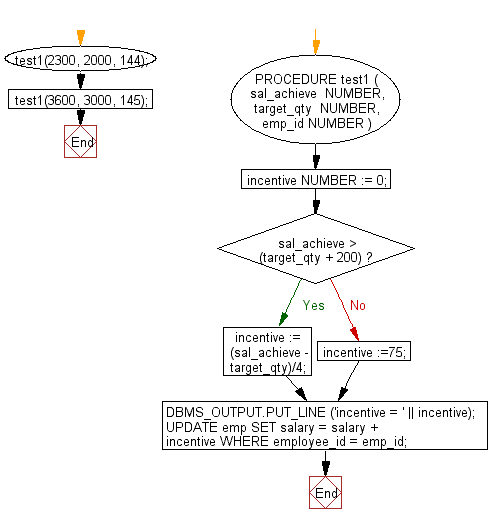﻿ PL/SQL Control Statement: Calculate the incentive on a specific target otherwise a general incentive to be paid using IF-THEN-ELSE - w3resource# PL/SQL Control Statement Exercises: Calculate the incentive on a specific target otherwise a general incentive to be paid using IF-THEN-ELSE

## PL/SQL Control Statement: Exercise-4 with Solution

Write a PL/SQL procedure to calculate the incentive on a specific target otherwise a general incentive to be paid using IF-THEN-ELSE.

Sample Solution:

PL/SQL Code:

``````DECLARE
PROCEDURE test1 (
sal_achieve  NUMBER,
target_qty  NUMBER,
emp_id NUMBER
)
IS
incentive   NUMBER := 0;

BEGIN
IF sal_achieve > (target_qty + 200) THEN
incentive := (sal_achieve - target_qty)/4;
ELSE
incentive :=75;
END IF;
DBMS_OUTPUT.PUT_LINE ('incentive = ' || incentive);
UPDATE emp
SET salary = salary + incentive
WHERE employee_id = emp_id;

END test1;
BEGIN
test1(2300, 2000, 144);
test1(3600, 3000, 145);
END;
/
``````

Sample Output:

```incentive = 75
incentive = 150
```

Flowchart:Improve this sample solution and post your code through Disqus

What is the difficulty level of this exercise?

﻿